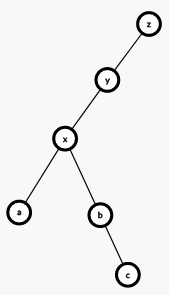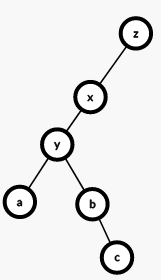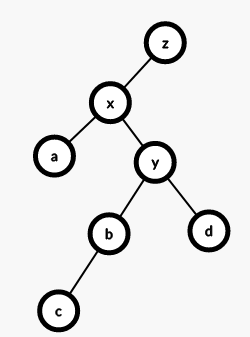# 前言

## 进入正题

$Splay$算法中，一个处处都要用到的东西就是旋转，即将当前节点与其前边一个节点依次旋转到目标位置。由于这个树是一个二叉搜索树，所以旋转之后要保证性质不变。我们就需要找到当前节点的父亲和爷爷节点，然后先更新爷爷节点与当前节点之间的关系，然后将父亲节点与该节点所属关系的另一个子树连起来，最后再处理一下该节点和父亲节点的关系。void rotate(int x){//旋转
int y=t[x].fa;
int z=t[y].fa;
int k=t[y].ch==x;//找到x是y的左还是右节点，便于进行上文中所说操作
t[z].ch[t[z].ch==y]=x;//以下都是上边说过的操作。
t[x].fa=z;
t[y].ch[k]=t[x].ch[k^1];
t[t[x].ch[k^1]].fa=y;
t[x].ch[k^1]=y;
t[y].fa=x;
pushup(y);
pushup(x);
}
void splay(int x,int goal){//旋转
while(t[x].fa!=goal){//父亲节点不是目标
int y=t[x].fa;
int z=t[y].fa;
if(z!=goal){//爷爷节点也不是目标
(t[y].ch==x)^(t[z].ch==y)?rotate(x):rotate(y);//其中一个点的左儿子是x就翻转x，不然翻转y（因为树有序，不能破坏性质）
}//上边这句话还需要细细钻研，本题解以后还会完善
rotate(x);
}
if(goal == 0){//到了根节点的话让根节点更新
root = x;
}
}


## 一些操作

struct Node
{
int ch;//子节点
int fa;//父节点
int cnt;//数量
int val;//值
int son;//儿子数量
}t[maxn];


### $1$、插入

void Add(int x){
int u=root,fa=0;
while(u&&t[u].val!=x){
fa=u;
u=t[u].ch[x>t[u].val];
}
if(u){//有的话直接大小加一
t[u].cnt++;
}
else{//没有该值的节点
u=++tot;
if(fa)t[fa].ch[x>t[fa].val]=u;
t[tot].ch=0;
t[tot].ch=0;
t[tot].val=x;
t[tot].fa=fa;
t[tot].cnt=1;
t[tot].son=1;
}
splay(u,0);
}


### $2$、查找值为$x$的排名：

void Find(int x){
int u=root;//从根开始
if(!u)return;//没有树就直接跳出
while(t[u].ch[x>t[u].val] && x!=t[u].val){//依次向下找到当前值的点
u=t[u].ch[x>t[u].val];//更新
}
splay(u,0);//旋转
}


### $3$、求前驱后继（这个操作求出来的是节点编号）

int Fr_last(int x,int flag){//前驱flag为0,后继为1
Find(x);//找到位置
int u=root;//根开始
if((t[u].val>x&&flag) || (t[u].val<x && !flag)){//当前点满足就直接返回
return u;
}
u=t[u].ch[flag];//向目标点（左或右）转移
while(t[u].ch[flag^1])u=t[u].ch[flag^1];//找到转移后最接近当前值的点
return u;//返回
}


### $4$、查找排名为$x$的值

int Find_thval(int x){
int u=root;//根开始
if(t[u].son<x){//如果没有这么多，直接返回0
return 0;
}
while(666666){//一直循环
int y=t[u].ch;//记录左儿子
if(x>t[y].son+t[u].cnt){//排名大就减去，然后走到右节点
x-=t[y].son+t[u].cnt;
u=t[u].ch;
}
else{
if(x<=t[y].son){//否则走到左节点
u=y;
}
else return t[u].val;//如果排名比上边的小，且比左节点的值大，这就是满足的价值，直接返回
}
}
}


### $5$、删除

void Delete(int x){
int Front=Fr_last(x,0);//前驱
int Last=Fr_last(x,1);//后继
splay(Front,0);//旋转
splay(Last,Front);
int del=t[Last].ch;//找到需要删除的点
if(t[del].cnt>1){//大于1直接减
t[del].cnt--;
splay(del,0);
}
else{//否则直接删除
t[Last].ch=0;
}
}


## 总结

### 板子题代码：

#include<bits/stdc++.h>
using namespace std;
const int maxn = 5e5+10;
const int Inf = 2147483647;
struct Node{
int son,ch,fa,cnt,val;
}t[maxn];
int n,tot,root;
void pushup(int x){
t[x].son = t[t[x].ch].son+t[t[x].ch].son+t[x].cnt;
}
void rotate(int x){
int y=t[x].fa;
int z=t[y].fa;
int k=t[y].ch==x;
t[z].ch[t[z].ch==y]=x;
t[x].fa=z;
t[y].ch[k]=t[x].ch[k^1];
t[t[x].ch[k^1]].fa=y;
t[x].ch[k^1]=y;
t[y].fa=x;
pushup(y);
pushup(x);
}
void splay(int x,int goal){
while(t[x].fa!=goal){
int y=t[x].fa;
int z=t[y].fa;
if(z!=goal){
(t[y].ch==x)^(t[z].ch==y)?rotate(x):rotate(y);
}
rotate(x);
}
if(goal == 0){
root = x;
}
}
void Find(int x){
int u=root;
if(!u)return;
while(t[u].ch[x>t[u].val] && x!=t[u].val){
u=t[u].ch[x>t[u].val];
}
splay(u,0);
}

int u=root,fa=0;
while(u&&t[u].val!=x){
fa=u;
u=t[u].ch[x>t[u].val];
}
if(u){
t[u].cnt++;
}
else{
u=++tot;
if(fa)t[fa].ch[x>t[fa].val]=u;
t[tot].ch=0;
t[tot].ch=0;
t[tot].val=x;
t[tot].fa=fa;
t[tot].cnt=1;
t[tot].son=1;
}
splay(u,0);
}
int Fr_last(int x,int flag){
Find(x);
int u=root;
if((t[u].val>x&&flag) || (t[u].val<x && !flag)){
return u;
}
u=t[u].ch[flag];
while(t[u].ch[flag^1])u=t[u].ch[flag^1];
return u;
}
void Delete(int x){
int Front=Fr_last(x,0);
int Last=Fr_last(x,1);
splay(Front,0);
splay(Last,Front);
int del=t[Last].ch;
if(t[del].cnt>1){
t[del].cnt--;
splay(del,0);
}
else{
t[Last].ch=0;
}
}
int Find_thval(int x){
int u=root;
if(t[u].son<x){
return 0;
}
while(666666){
int y=t[u].ch;
if(x>t[y].son+t[u].cnt){
x-=t[y].son+t[u].cnt;
u=t[u].ch;
}
else{
if(x<=t[y].son){
u=y;
}
else return t[u].val;
}
}
}
int main(){
int n;
scanf("%d",&n);
while(n--){
int opt;
scanf("%d",&opt);
int x;
if(opt == 1){
scanf("%d",&x);
}
if(opt == 2){
scanf("%d",&x);
Delete(x);
}
if(opt == 3){
scanf("%d",&x);
Find(x);
int ans = t[t[root].ch].son;
printf("%d\n",ans);
}
if(opt == 4){
int ans;
scanf("%d",&x);
ans = Find_thval(x+1);
printf("%d\n",ans);
}
if(opt == 5){
scanf("%d",&x);
int ans = Fr_last(x,0);
printf("%d\n",t[ans].val);
}
if(opt == 6){
scanf("%d",&x);
int ans = Fr_last(x,1);
printf("%d\n",t[ans].val);
}
}
return 0;
}

posted @ 2020-07-10 20:37  Vocanda  阅读(131)  评论(4编辑  收藏